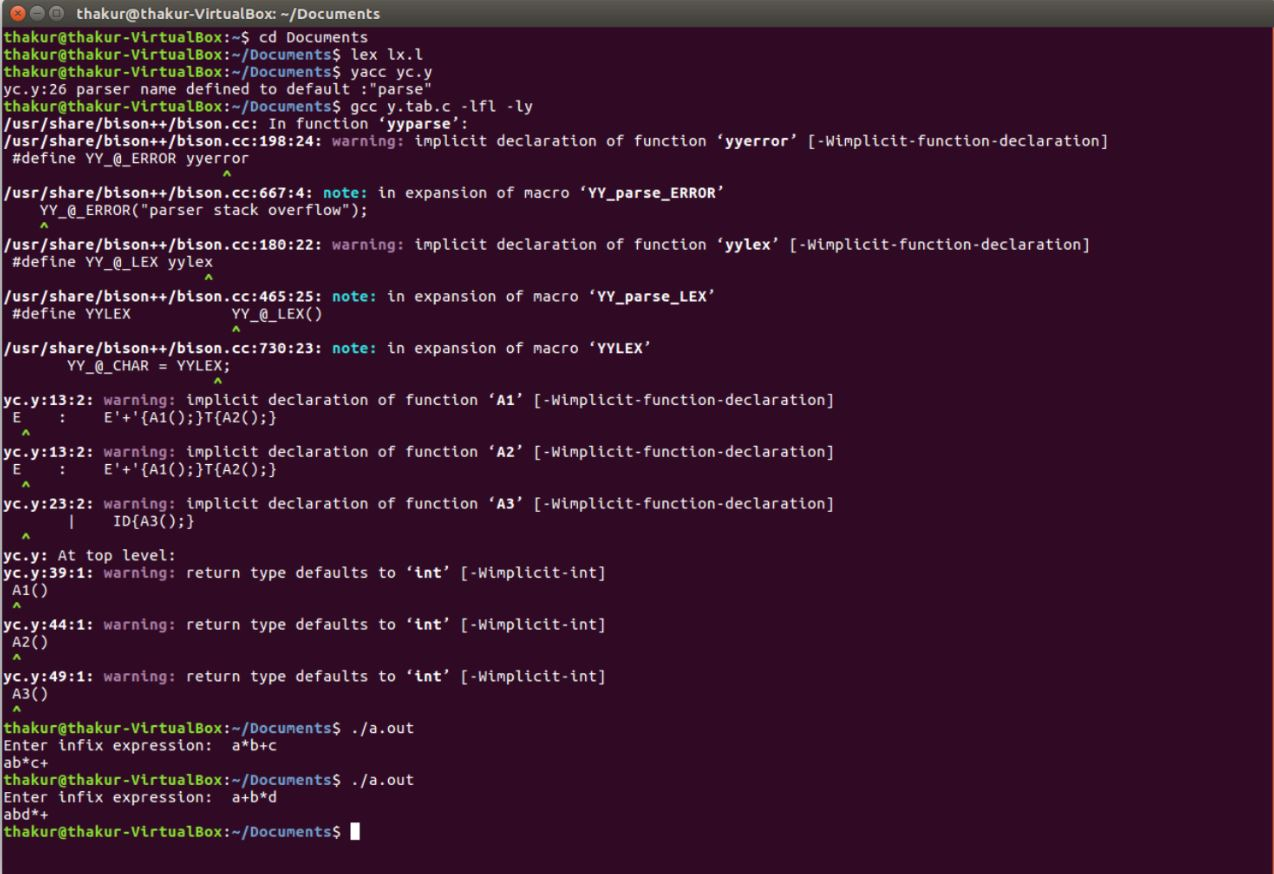# YACC program for Conversion of Infix to Postfix expression

Problem: Write a YACC program for conversion of Infix to Postfix expression.

Explanation:
YACC (Yet another Compiler-Compiler) is the standard parser generator for the Unix operating system. An open source program, yacc generates code for the parser in the C programming language. The acronym is usually rendered in lowercase but is occasionally seen as YACC or Yacc.

Examples:

```Input: a*b+c
Output: ab*c+

Input: a+b*d
Output: abd*+ ```

Lexical Analyzer Source Code:

 `%{ ` `  ``/* Definition section */` `%} ` `ALPHA [A-Z a-z] ` `DIGIT [0-9] ` ` `  `/* Rule Section */` `%% ` `{ALPHA}({ALPHA}|{DIGIT})*  ``return` `ID; ` `{DIGIT}+                   {yylval=``atoi``(yytext); ``return` `ID;} ` `[\n \t]                    yyterminate(); ` `.                          ``return` `yytext; ` `%% `

Parser Source Code:

 `%{ ` `   ``/* Definition section */` `   ``#include ` `   ``#include ` `%} ` ` `  `%token    ID ` `%left    ``'+'` `'-'` `%left    ``'*'` `'/'` `%left    UMINUS ` ` `  `/* Rule Section */` `%% ` ` `  `S  :  E ` `E  :  E``'+'``{A1();}T{A2();} ` `   ``|  E``'-'``{A1();}T{A2();} ` `   ``|  T ` `   ``; ` `T  :  T``'*'``{A1();}F{A2();} ` `   ``|  T``'/'``{A1();}F{A2();} ` `   ``|  F ` `   ``; ` `F  :  ``'('``E{A2();}``')'` `   ``|  ``'-'``{A1();}F{A2();} ` `   ``|  ID{A3();} ` `   ``; ` ` `  `%% ` ` `  `#include"lex.yy.c" ` `char` `st; ` `int` `top=0; ` ` `  `//driver code ` `int` `main() ` `{ ` `    ``printf``(``"Enter infix expression:  "``);  ` `    ``yyparse(); ` `    ``printf``(``"\n"``); ` `    ``return` `0; ` `} ` `A1() ` `{ ` `    ``st[top++]=yytext; ` `} ` ` `  `A2() ` `{ ` `    ``printf``(``"%c"``, st[--top]); ` `} ` ` `  `A3() ` `{ ` `    ``printf``(``"%c"``, yytext); ` `} `

Output:Whether you're preparing for your first job interview or aiming to upskill in this ever-evolving tech landscape, GeeksforGeeks Courses are your key to success. We provide top-quality content at affordable prices, all geared towards accelerating your growth in a time-bound manner. Join the millions we've already empowered, and we're here to do the same for you. Don't miss out - check it out now!

Previous
Next